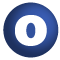# EOF

#### object EOF extends Token with Product

A class for end-of-file tokens

definition classes: Tokens

1. Product
2. Equals
3. Token
4. AnyRef
5. Any

### Value Members

1. #### def canEqual(arg0: Any): Boolean

A method that should be called from every well-designed equals method that is open to be overridden in a subclass

A method that should be called from every well-designed equals method that is open to be overridden in a subclass. See Programming in Scala, Chapter 28 for discussion and design.

definition classes: EOFEquals

3. #### def equals(arg0: Any): Boolean

This method is used to compare the receiver object (`this`) with the argument object (`arg0`) for equivalence

This method is used to compare the receiver object (`this`) with the argument object (`arg0`) for equivalence.

The default implementations of this method is an equivalence relation:

• It is reflexive: for any instance `x` of type `Any`, `x.equals(x)` should return `true`.
• It is symmetric: for any instances `x` and `y` of type `Any`, `x.equals(y)` should return `true` if and only if `y.equals(x)` returns `true`.
• It is transitive: for any instances `x`, `y`, and `z` of type `AnyRef` if `x.equals(y)` returns `true` and `y.equals(z)` returns `true`, then `x.equals(z)` should return `true`.

If you override this method, you should verify that your implementation remains an equivalence relation. Additionally, when overriding this method it is often necessary to override `hashCode` to ensure that objects that are "equal" (`o1.equals(o2)` returns `true`) hash to the same `Int` (`o1.hashCode.equals(o2.hashCode)`).

arg0

the object to compare against this object for equality.

returns

`true` if the receiver object is equivalent to the argument; `false` otherwise.

definition classes: AnyRef ⇐ Any
4. #### def hashCode(): Int

Returns a hash code value for the object

Returns a hash code value for the object.

The default hashing algorithm is platform dependent.

Note that it is allowed for two objects to have identical hash codes (`o1.hashCode.equals(o2.hashCode)`) yet not be equal (`o1.equals(o2)` returns `false`). A degenerate implementation could always return `0`. However, it is required that if two objects are equal (`o1.equals(o2)` returns `true`) that they have identical hash codes (`o1.hashCode.equals(o2.hashCode)`). Therefore, when overriding this method, be sure to verify that the behavior is consistent with the `equals` method.

definition classes: AnyRef ⇐ Any
5. #### def productArity: Int

return k for a product `A(x_1,`

``` return k for a product A(x_1,...,x_k) definition classes: EOF ⇐ Product ```
``` ```
6. ``` def productElement(arg0: Int): Any for a product A(x_1, for a product A(x_1,...,x_k), returns x_(n+1) for 0 <= n < k definition classes: EOF ⇐ Product ```
7. ``` def productElements: Iterator[Any] ```
8. ``` def productIterator: Iterator[Any] An iterator that returns all fields of this product An iterator that returns all fields of this product definition classes: Product ```
9. ``` def productPrefix: String By default the empty string By default the empty string. Implementations may override this method in order to prepend a string prefix to the result of the toString methods. definition classes: EOF ⇐ Product ```
``` ```
``` ```
``` ```